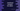# How to compare two dates in JavaScript# Introduction :

Date comparison is required any time you are using Date in your code. In Javascript, we can easily compare two Date objects. In this tutorial, I will show you how to compare two Date with one example.

## Date comparison :

Javascript Date provides one method called getTime() that returns the number of milliseconds since Jan 1, 1970, 00:00:00.000 GMT. For example :

``````var date = new Date();
console.log(date.getTime());

setTimeout(()=>{
var date1 = new Date();
console.log(date1.getTime());
},500);``````

It will print something like below :

``````1569511747222
1569511747730``````

As you have seen above, date and date1 variables are initialized at different time and that’s why getTime() is returning different values for both.

This is a numeric value and if we want to compare two Date, we can simply do it by comparing the values returning by the getTime() method.

## Javascript program :

``````var firstDate = new Date("12-09-2012");
var secondDate = new Date("11-01-2011");

if (firstDate.getDate() > secondDate.getDate()) {
console.log(`firstDate is larger than secondDate.`)
} else {
console.log(`firstDate is smaller than secondDate.`)
}``````

It will print the below output :

``firstDate is larger than secondDate.``